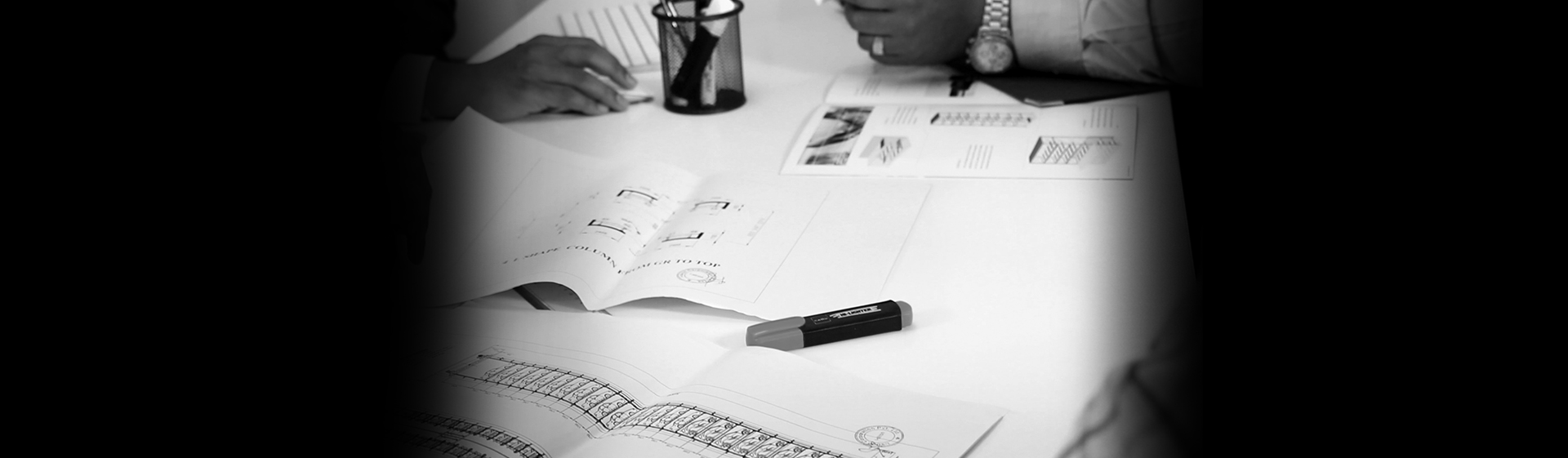Home  >  Product Dimension Calculator

## Product Dimension Calculator

Reset Product Dimension Calculator

### 2DS

Total width (W1) required is 0
Total length (L1) required is 0
Platform length (L2) is 0
Clear height (H) required is 0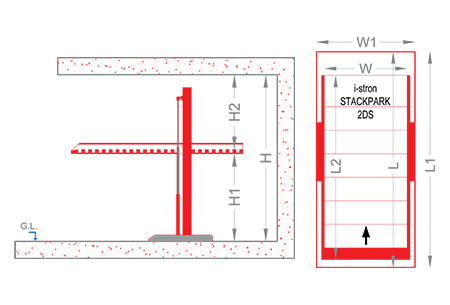##### Height
• Entry car height (H1)
0
• Upper car height (H2)
0
• Clear height (H) required is
0
##### Width
• Clear platform width (W)
0
• Total width (W1) required is
0
##### Length
• Car length (L)
0
• Total length (L1) required is
0
• Platform length (L2) is
0

### 3DS

Total width (W1) required is 0
Total length (L1) required is 0
Platform length (L2) is 0
Clear height (H) required is 0##### Height
• Entry car height (H1)
0
• Middle car height (H2)
0
• Top car height (H3)
0
• Clear height (H) required is
0
##### Width
• Clear platform width (W)
0
• Total width (W1) required is
0
##### Length
• Car length (L)
0
• Total length (L1) required is
0
• Platform length (L2) is
0

### Incline

Total width (W1) required is 0
Total length (L1) required is 0
Platform length (L2) is 0
Clear height (H) required is 0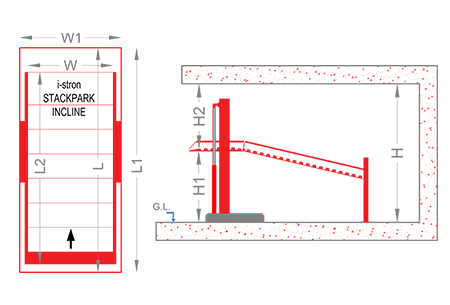##### Height
• Entry car height (H1)
0
• Upper car height (H2)
0
• Clear height (H) required is
0
##### Width
• Clear platform width (W)
0
• Total width (W1) required is
0
##### Length
• Car length (L)
0
• Total length (L1) required is
0
• Platform length (L2) is
0

### Cantilever

Total width (W1) required is 0
Total length (L1) required is 0
Platform length (L2) is 0
Clear height (H) required is 0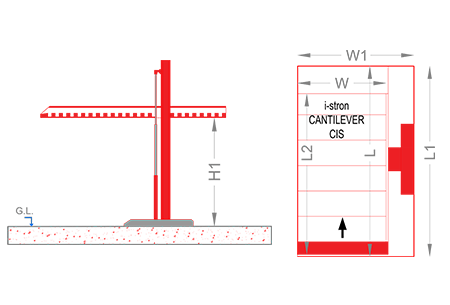##### Height
• Entry car height (H1)
0
• Upper car height (H2)
0
• Clear height (H) required is
0
##### Width
• Clear platform width (W)
0
• Total width (W1) required is
0
##### Length
• Car length (L)
0
• Total length (L1) required is
0
• Platform length (L2) is
0

### 2IP

Total width (W1) required is 0
Total length (L1) required is 0
Platform length (L2) is 0
Clear height (H) required is 0
Pit depth (P) required is 0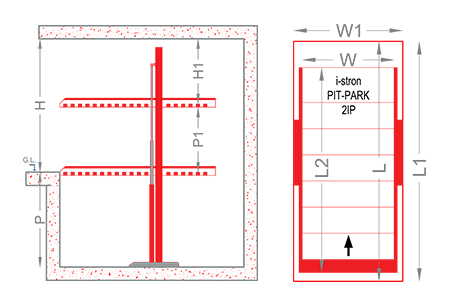##### Height
• Entry car height (H1)
0
• lower car height (P1)
0
• Clear height (H) required is
0
• Pit depth (P) required is
0
##### Width
• Clear platform width (W)
0
• Total width (W1) required is
0
##### Length
• Car length (L)
0
• Total length (L1) required is
0
• Platform length (L2) is
0

### 2DP

Total width (W1) required is 0
Total length (L1) required is 0
Platform length (L2) is 0
Clear height (H) required is 0
Pit depth (P) required is 0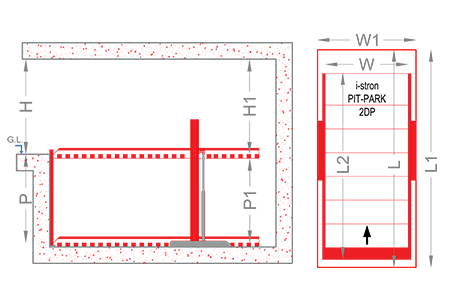##### Height
• Entry car height (H1)
0
• Lower car height (P1)
0
• Clear height (H) required is
0
• Pit depth (P) required is
0
##### Width
• Clear platform width (W)
0
• Total width (W1) required is
0
##### Length
• Car length (L)
0
• Total length (L1) required is
0
• Platform length (L2) is
0

### 3DP

Total width (W1) required is 0
Total length (L1) required is 0
Platform length (L2) is 0
Clear height (H) required is 0
Pit depth (P) required is 0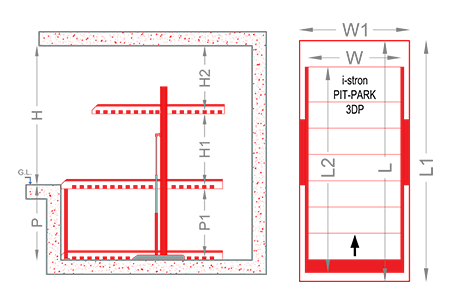##### Height
• Entry car height (H1)
0
• Upper car height (H2)
0
• Clear height (H) required is
0
• Lower car height (P1)
0
• Pit depth (P) required is
0
##### Width
• Clear platform width (W)
0
• Total width (W1) required is
0
##### Length
• Car length (L)
0
• Total length (L1) required is
0
• Platform length (L2) is
0

### 3IP

Total width (W1) required is 0
Total length (L1) required is 0
Platform length (L2) is 0
Clear height (H) required is 0
Pit depth (P) required is 0##### Height
• Entry car height (H1)
0
• Middle car height (P1)
0
• Lower car height (P2)
0
• Clear height (H) required is
0
• Pit depth (P) required is
0
##### Width
• Clear platform width (W)
0
• Total width (W1) required is
0
##### Length
• Car length (L)
0
• Total length (L1) required is
0
• Platform length (L2) is
0

### 3RP

Total width (W1) required is 0
Total length (L1) required is 0
Platform length (L2) is 0
Clear height (H) required is 0
Pit depth (P) required is 0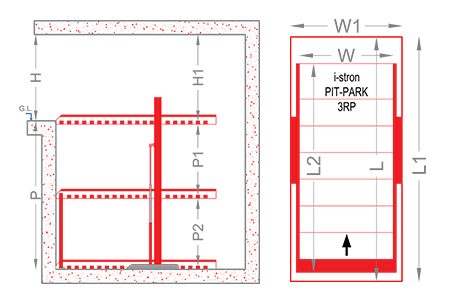##### Height
• Entry car height (H1)
0
• Middle car height (P1)
0
• Lower car height (P2)
0
• Clear height (H) required is
0
• Pit depth (P) required is
0
##### Width
• Clear platform width (W)
0
• Total width (W1) required is
0
##### Length
• Car length (L)
0
• Total length (L1) required is
0
• Platform length (L2) is
0

### 2IC

Total Platform is 00
•
•
•
•
•
•
•
•
•
•
•
•
•
•
•
•
•
•
•
•
Clear platform width required is 0
Total width (W1) required is 0
Total length (L1) required is 0
Platform length (L2) is 0
Clear height (H) required is 0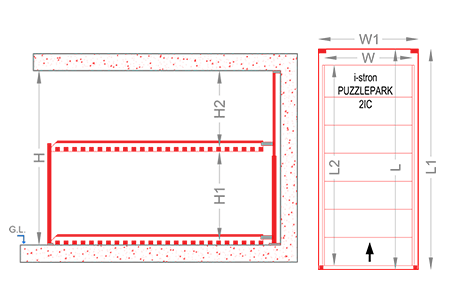##### Height
• Entry car height (H1)
0
• Upper car height (H2)
0
• Clear height (H) required is
0
##### Width
• Clear platform width (W)
0
• Total width (W1) required is
0
##### Length
• Car length (L)
0
• Total length (L1) required is
0
• Platform length (L2) is
0

### 2HC

Total Platform is 00
•
•
•
•
•
•
•
•
•
•
•
•
•
•
•
•
•
•
•
•
Clear platform width required is 0
Total width (W1) required is 0
Total length (L1) required is 0
Platform length (L2) is 0
Clear height (H) required is 0
Pit depth (P) required is 0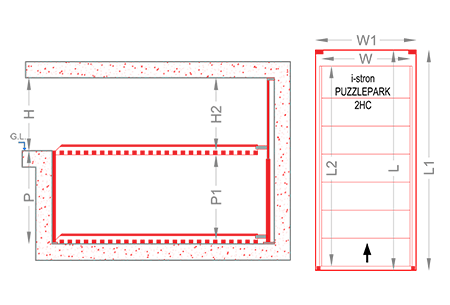##### Height
• Entry car height (H1)
0
• Clear height (H) required is
0
• Pit (P2) is
0
• Pit depth (P) required is
0
##### Width
• Clear platform width (W)
0
• Total width (W1) required is
0
##### Length
• Car length (L)
0
• Total length (L1) required is
0
• Platform length (L2) is
0

### 3IC

Total Platform is 00
•
•
•
•
•
•
•
•
•
•
•
•
•
•
•
•
•
•
•
•
•
•
•
•
•
•
•
•
•
•
Clear platform width required is 0
Total width (W1) required is 0
Total length (L1) required is 0
Platform length (L2) is 0
Clear height (H) required is 0
Pit depth (P) required is 0##### Height
• Entry car height (H1)
0
• Upper car height (H2)
0
• Clear height (H) required is
0
• Pit (P1)
0
• Pit depth (P) required is
0
##### Width
• Clear platform width (W)
0
• Total width (W1) required is
0
##### Length
• Car length (L)
0
• Total length (L1) required is
0
• Platform length (L2) is
0

### 2TC

Total Platform is 00
•
•
•
•
•
•
•
•
•
•
•
•
•
•
•
•
•
•
•
•
Clear platform width required is 0
Total width (W1) required is 0
Total length (L1) required is 0
Platform length (L2) is 0
Clear height (H) required is 0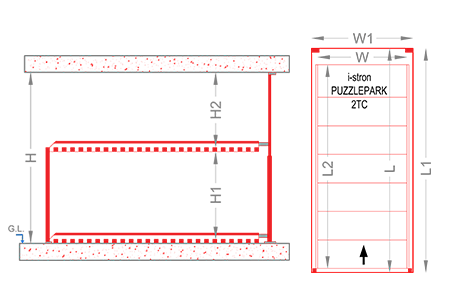##### Height
• Lower car height (H1)
0
• Upper car height (H2)
0
• Clear height (H) required is
0
##### Width
• Clear platform width (W)
0
• Total width (W1) required is
0
##### Length
• Car length (L)
0
• Total length (L1) required is
0
• Platform length (L2) is
0

### 3TC

Total Platform is 00
•
•
•
•
•
•
•
•
•
•
•
•
•
•
•
•
•
•
•
•
•
•
•
•
•
•
•
•
•
•
Clear platform width required is 0
Total width (W1) required is 0
Upper platform length (L1) required is 0
Middle platform length (L2) required is 0
Lower platform length (L3) required is 0
Clear height (H) required is 0
Clear pit (P1) required is 0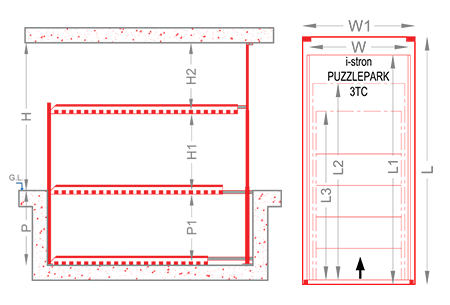##### Height
• Entry car height (H1)
0
• Entry upper height (H2)
0
• Clear height (H)
0
• Pit (P1) required is
0
##### Width
• Clear platform width (W)
0
• Total width (W1) required is
0
##### Length
• Upper platform length (L1)
0
• Middle platform length (L2)
0
• Lower platform length (L3)
0

### Puzzle

Total width (W) required per grid is 0
Total width required for the system is 0
No of Levels to be allotted for SUVs above ground is 0
No of Levels to be allotted for SUVs in Pit is 0
No of Levels to be allotted for Sedans above ground is 0
No of Levels to be allotted for Sedans in Pit is 0
Total Height Required is 0
Total Pit depth Required is 0
Total Length Required 0##### Height
• No of Levels for SUVs above pit is
0
• No of Level for SUVs in Pit is
0
• No of Levels for Sedans above pit is
0
• No of Level for Sedans in Pit is
0
• Total Height Required for the system is
0
• Total Pit Depth Required For the System is
0
##### Width
• Clear Platform Width is
0
• Total Width Per Grid is
0
• Total System Width is
0
##### Length
• Car Length is
0
• Total Length Required is
0

### Tower

Total width (W) & length (L) required is 0
Number of SUV is 0
Number of Sedan is 0
Total Height (H) is 0
Pit depth (P) required is 1500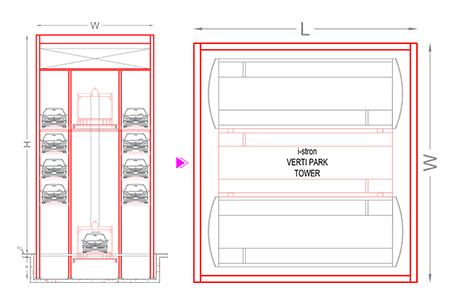##### Height
• Number of Cars
0
• Number of SUV
0
• Number of Sedan
0
• Total Height (H) is
0
• Pit depth (P) required is
1500
##### Width & Length
• Clear width (W)
0
• Clear Length (L)
0
• Total width & length required is
0